# Physics of Everyday Phenomena W Thomas Griffith Juliet

• Slides: 18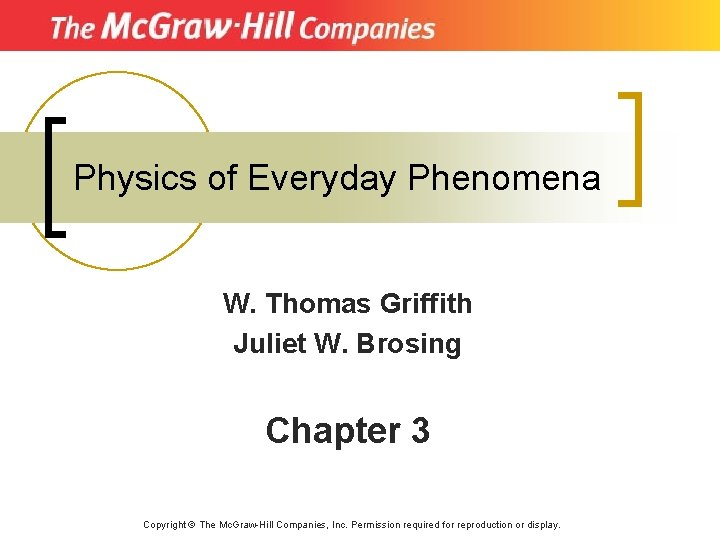Physics of Everyday Phenomena W. Thomas Griffith Juliet W. Brosing Chapter 3 Copyright © The Mc. Graw-Hill Companies, Inc. Permission required for reproduction or display.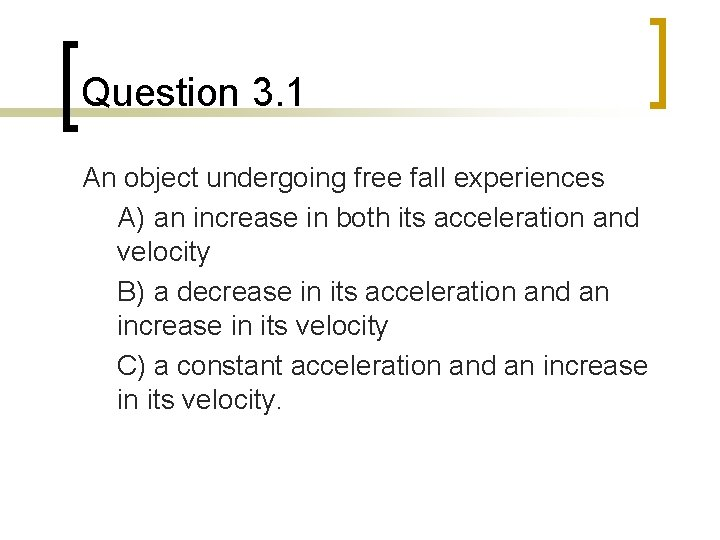Question 3. 1 An object undergoing free fall experiences A) an increase in both its acceleration and velocity B) a decrease in its acceleration and an increase in its velocity C) a constant acceleration and an increase in its velocity.Question 3. 2 If a feather and a brick are both dropped from the same height at the same time in the classroom A) both experience the same acceleration due to gravity B) the net acceleration of both is the same C) the net acceleration of the feather is greater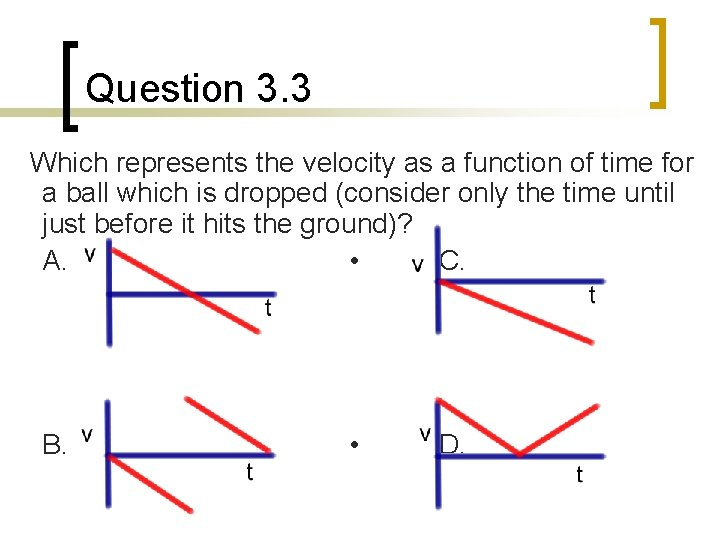Question 3. 3 Which represents the velocity as a function of time for a ball which is dropped (consider only the time until just before it hits the ground)? A. • C. B. • D.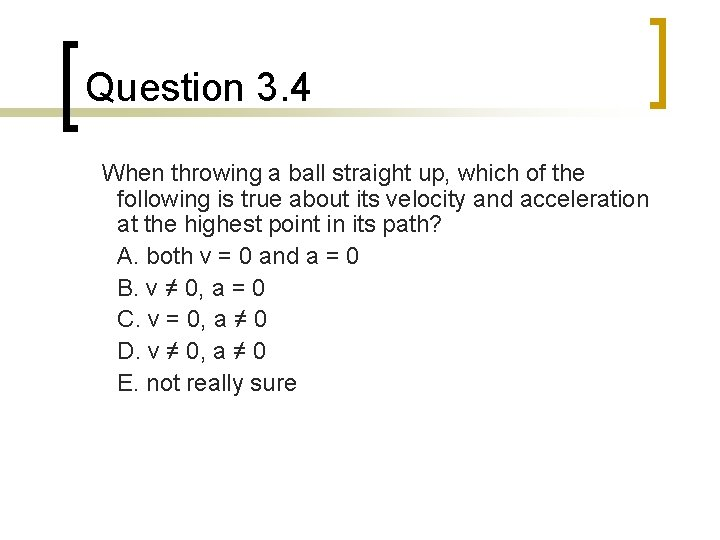Question 3. 4 When throwing a ball straight up, which of the following is true about its velocity and acceleration at the highest point in its path? A. both v = 0 and a = 0 B. v ≠ 0, a = 0 C. v = 0, a ≠ 0 D. v ≠ 0, a ≠ 0 E. not really sure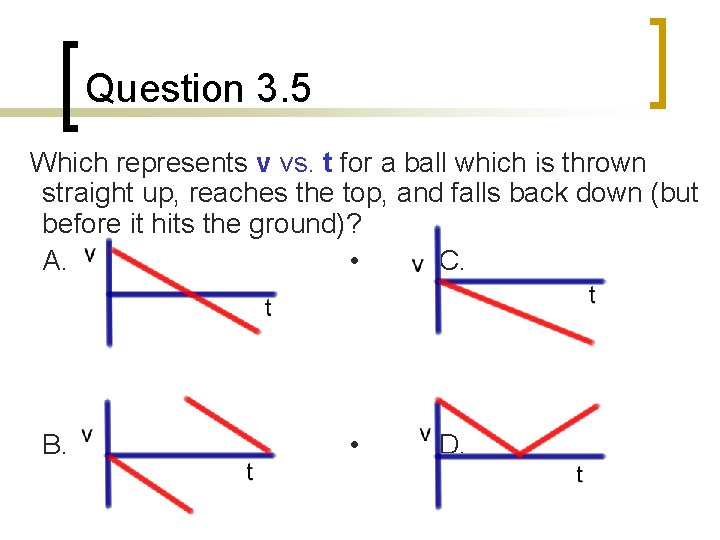Question 3. 5 Which represents v vs. t for a ball which is thrown straight up, reaches the top, and falls back down (but before it hits the ground)? A. • C. B. • D.Question 3. 6 Alec and Michelle release their snowballs from the same height, at the same time and with the same speed. Alec's is thrown upward while Michelle’s is thrown downward. Which one hits the ground with the greatest speed (ignore air resistance)? A. the one thrown upward B. the one thrown downward C. they hit with the same speed D. it depends on the initial height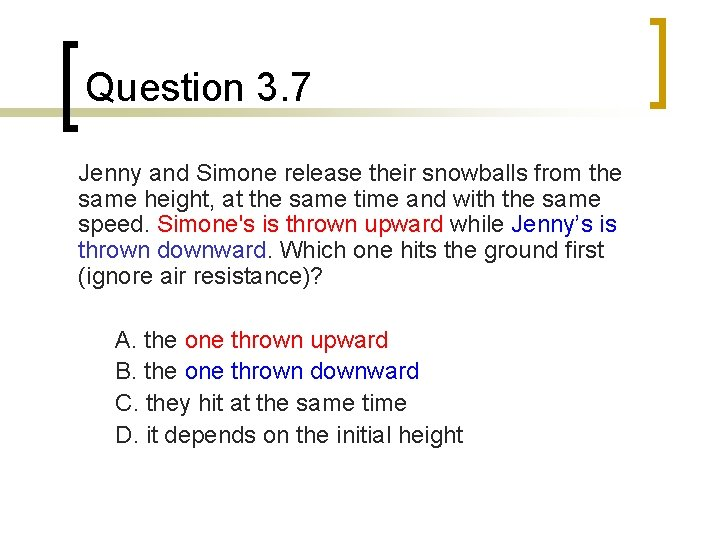Question 3. 7 Jenny and Simone release their snowballs from the same height, at the same time and with the same speed. Simone's is thrown upward while Jenny’s is thrown downward. Which one hits the ground first (ignore air resistance)? A. the one thrown upward B. the one thrown downward C. they hit at the same time D. it depends on the initial heightQuestion 3. 8 The directions of the velocity and acceleration of an object which is thrown straight upward and then falls back down (ignore the effects of air resistance) have the following characteristics: A. the velocity and acceleration are always directed downward B. the velocity is always upward but the acceleration changes direction C. the velocity is always upward and the acceleration is always downward D. the velocity changes direction but the acceleration always stays the same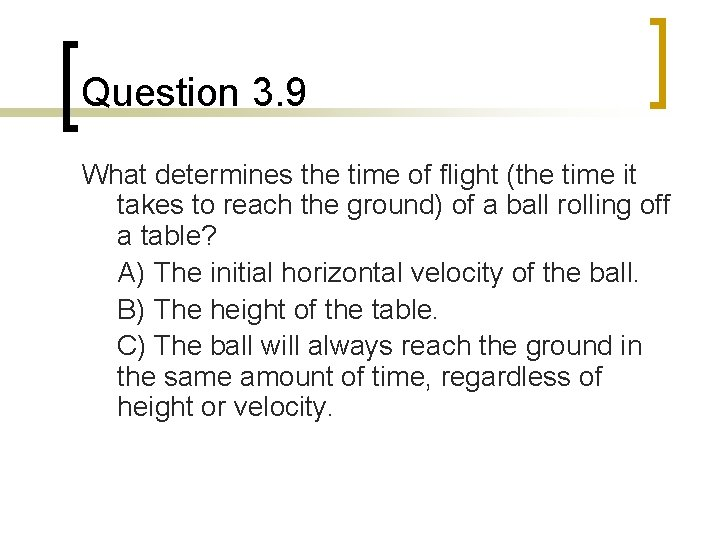Question 3. 9 What determines the time of flight (the time it takes to reach the ground) of a ball rolling off a table? A) The initial horizontal velocity of the ball. B) The height of the table. C) The ball will always reach the ground in the same amount of time, regardless of height or velocity.Question 3. 10 Alyssa throws a snowball horizontally from the top of a roof; Ryan throws a snowball straight down from the same point. Once in flight, the acceleration of Alyssa’s snowball is _____ the acceleration of Ryan’s snowball. A. greater than B. less than C. equal toQuestion 3. 11 Anders and Taisen release their snowballs from the same height and at the same time. Ander's is dropped while Taisen’s is thrown horizontally. Which one hits the ground first? A. the "dropped" snowball B. the "thrown" snowball C. they hit at the same time D. it depends on how hard Boris threw E. it depends on the initial height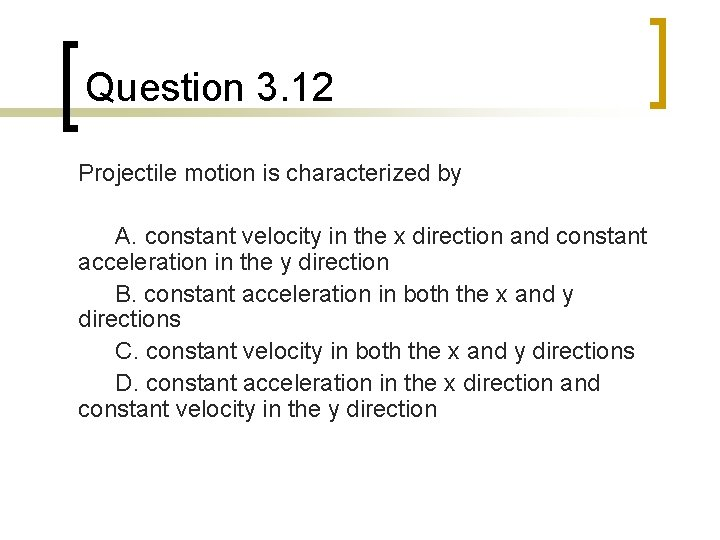Question 3. 12 Projectile motion is characterized by A. constant velocity in the x direction and constant acceleration in the y direction B. constant acceleration in both the x and y directions C. constant velocity in both the x and y directions D. constant acceleration in the x direction and constant velocity in the y directionQuestion 3. 13 In projectile motion the horizontal component of the velocity at the top of the trajectory is A) non-zero, and less than the initial horizontal component of the velocity. B) non-zero, and equal to the initial horizontal component of the velocity C) zero D) non-zero, and greater than the initial horizontal component of the velocity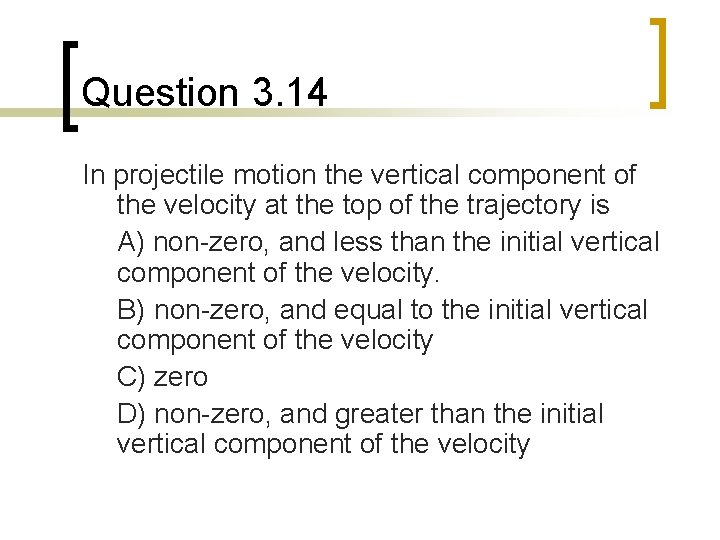Question 3. 14 In projectile motion the vertical component of the velocity at the top of the trajectory is A) non-zero, and less than the initial vertical component of the velocity. B) non-zero, and equal to the initial vertical component of the velocity C) zero D) non-zero, and greater than the initial vertical component of the velocityQuestion 3. 15 In projectile motion the velocity at the top of the trajectory is A) zero B) non-zero, since the x component of the velocity has remained constant. C) larger than at any other time during the trajectory.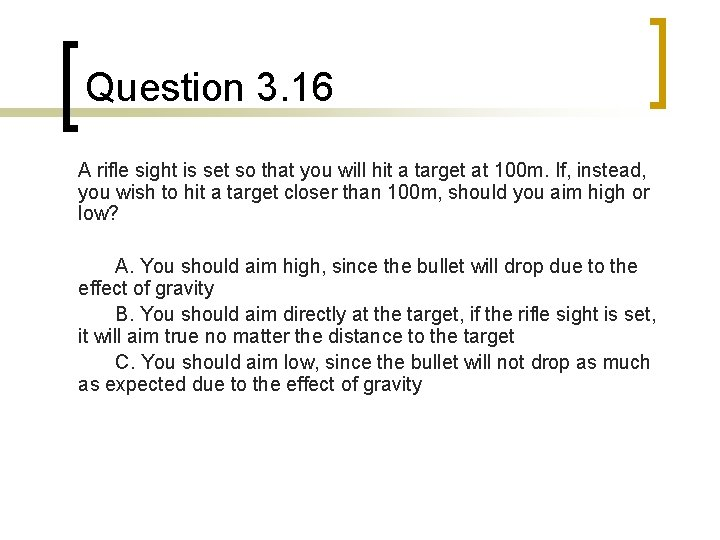Question 3. 16 A rifle sight is set so that you will hit a target at 100 m. If, instead, you wish to hit a target closer than 100 m, should you aim high or low? A. You should aim high, since the bullet will drop due to the effect of gravity B. You should aim directly at the target, if the rifle sight is set, it will aim true no matter the distance to the target C. You should aim low, since the bullet will not drop as much as expected due to the effect of gravity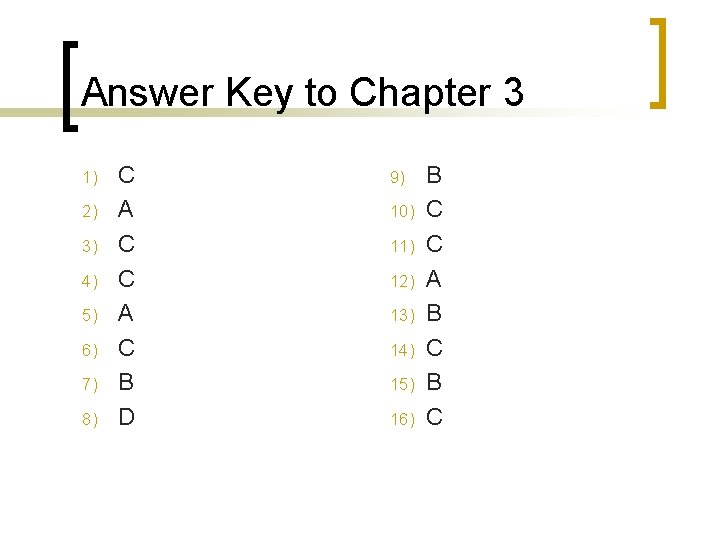Answer Key to Chapter 3 1) 2) 3) 4) 5) 6) 7) 8) C A C B D 9) 10) 11) 12) 13) 14) 15) 16) B C C A B C